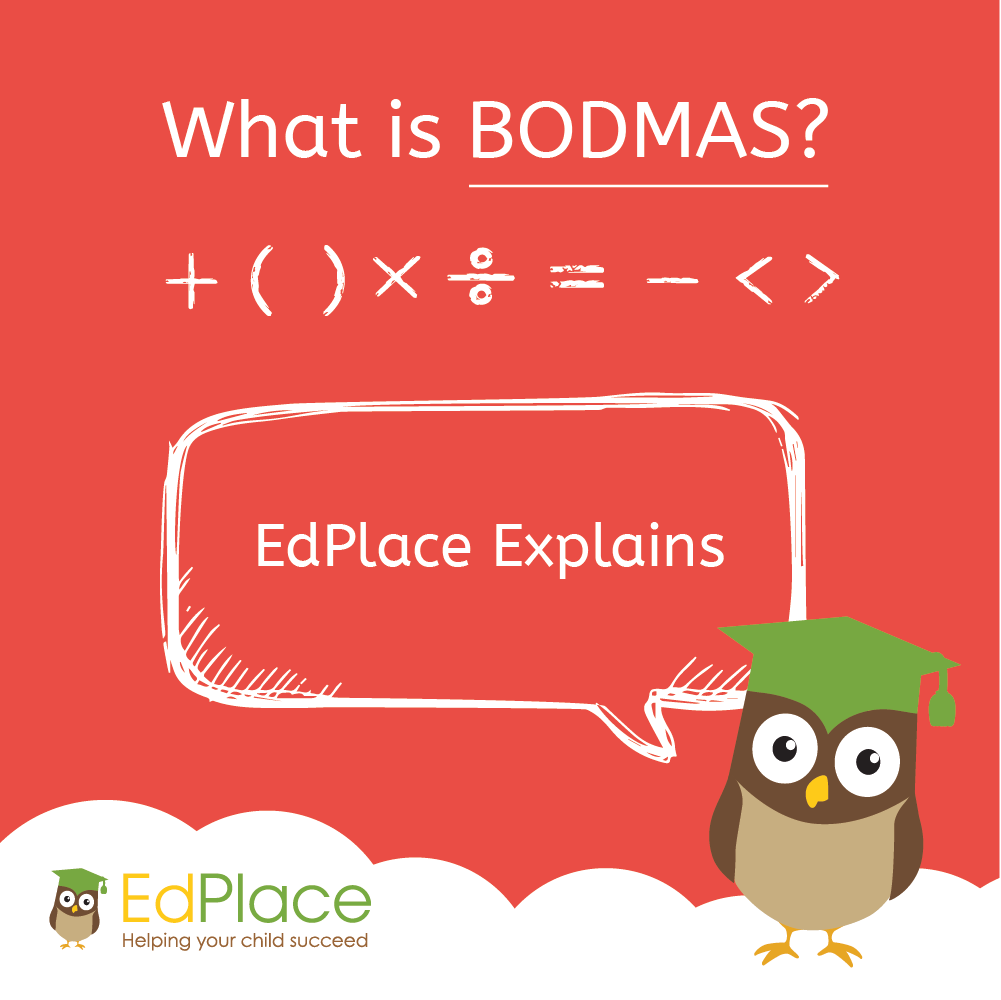# What is BODMAS?

BODMAS (or sometimes BIDMAS) is a simple acronym that helps you remember the order in which to solve calculations. Sometimes calculations can get a little more complicated than 2+2! For example, what would you do if you were given a calculation like 3 x (4 x 5²) ÷ 6 + 7 – 8 =? The meaning of BODMAS is important to make sure you get answers to questions like these right; without BODMAS explained to you properly, it’s likely you’ll get more questions wrong on complicated maths worksheets!But what does BODMAS stand for?

BODMAS Meaning

So what is BODMAS? - Don’t Panic! BODMAS is here to help. BODMAS stands for:

 Brackets Orders or                   Indices Division Multiplication Addition Subtraction Calculations inside brackets always come first This means any indices, powers or roots go next Multiply or divide before you add or subtract. If there is division and multiplication, complete from left to right. Finally, complete any addition and subtraction from left to right.

If you don’t get the BODMAS order correct, you’ll probably end up with a completely different answer, so make sure you get remembering!

# BODMAS Explained

Maths teacher, Alison explains:

BODMAS/BIDMAS is important to remember; if the order is wrong, your answer will be too! Below, we have some examples of BODMAS questions to help you test your understanding of the theory! Don’t worry if you don’t get it straight away - BODMAS sums are hard!

So, let's calculate the BODMAS exercise above:

3 x (4 x 5²) ÷ 6 + 7 – 8 = ?

## Steps:

### () Brackets

Complete the calculation inside the brackets first.

4 x 5² = Work out 5 squared first (Orders)

5² = 25

So 4 x 25 = 100

Our calculation now becomes 3 x 100 ÷ 6 + 7 – 8 = ?

### ÷× Division and Multiplication

In this calculation, we have both division and multiplication.

Follow the calculation left to right

3 x 100 = 300

300 ÷ 6 = 50

Our calculation now becomes 50 + 7 – 8 = ?

### 3.

In this calculation, we have both addition and subtraction.

Follow the calculation left to right

50 + 7 = 57

57 – 8 = 49

# Easy Ways to Remember BODMAS/BIDMAS

Brackets first then Orders (or sometimes Indices).

Division and/or Multiplication left to right followed by Addition and/or Subtraction left to right.

# BODMAS Botch-ups

The BODMAS rule in maths isn’t always easy to understand - here are a few examples of BODMAS and easy mistakes many students make. Can you spot their errors and fix them? Answers at the bottom of the page

 Question 1 4 + 3 x 2                     = 7 x 2 = 14 Question 2 12 – 4 ÷ 2                     = 8 ÷ 2 = 4 Question 3 5²– 6                     = 10 – 6 = 4 Question 4 25 – 6 x 2                     6 x 2 = 12 12 – 25 = -13 = -13

# BODMAS Worksheets and Practice

It’s always good to practice your new skills!

Year 6:

Using BIDMAS – Order of operations in calculations

Year 7:

Using the order of operations in calculations - Worksheet 1

Using brackets on a calculator

Year 8:

Using brackets in different places for different answers

Using brackets on the calculator to work out combined operations

Multiplying out brackets

Multiplying out brackets and simplifying

Solve equations with brackets

Year 9:

Multiplying out brackets and simplifying

Multiplying out two brackets and simplifying

Using brackets on a calculator

# Answers to BODMAS Botch ups

 Question 1 4 + 3 x 2                     = 3 x 2 + 4 = 10 The student forgot to multiply first then add, instead they added first then multiplied. These are some great BIDMAS examples where things can go wrong. Question 2 12 – 4 ÷ 2                     = 4 ÷ 2 = 2 = 12 – 2 = 10 The student forgot to divide first then subtract, instead they subtracted first then divided. The best way to understand order of operations is to complete BIDMAS questions until you can remember it by heart. Question 3 5² – 6                     = 25 – 6 = 19 By not following the BIDMAS rule, the student doubled the 5 rather than multiplying it by itself. Question 4 25 – 6 x 2                     6 x 2 = 12 12 – 25 = -13 = -13 The student mixed up the subtraction. It should have been 25 – 12 not 12 – 25!WRITTEN BY: Ms Alison – ENGLISH TEACHER

••••